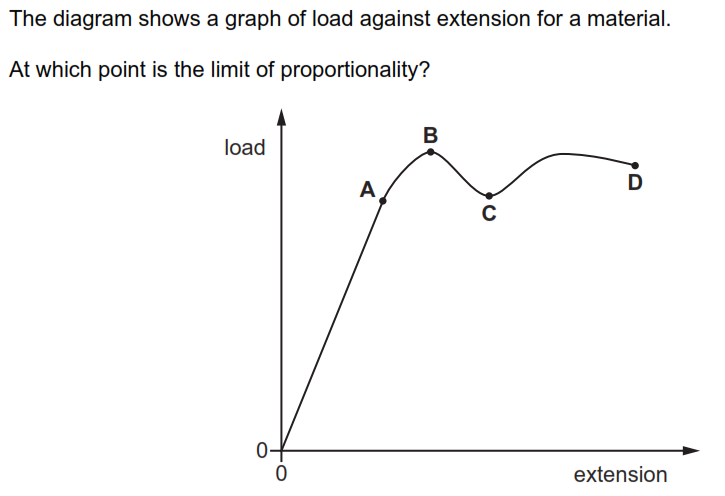# An Engineering Short Quiz About Deformation

10 Questions | Attempts: 288Settings.

• 1.
An unstretched elastic cord of length 15.0 cm stretches by 4.0 cm when a weight of 6.0 N is suspended from it. The cord does not exceed the limit of proportionality. What is the total length of the cord when the weight is 3.0 N?
• A.

2.0 cm

• B.

7.5 cm

• C.

9.5 cm

• D.

17.0 cm

• 2.
• A.

A

• B.

B

• C.

C

• D.

D

• 3.
• A.

A

• B.

B

• C.

C

• D.

D

• 4.
• A.

A

• B.

B

• C.

C

• D.

D

• 5.
Which object behaves as an elastic solid as it deforms?  A). A bullet as it hits a solid metal wall B). A car damaged in a collision C). A piece of metal cut by a saw D). A football as it is kicked
• A.

A

• B.

B

• C.

C

• D.

D

• 6.
• A.

A

• B.

B

• C.

C

• D.

D

• 7.
• A.

A

• B.

B

• C.

C

• D.

D

• 8.
• A.

Its base area and location of its centre of mass

• B.

Its weight and base area

• C.

Location of its centre of mass

• D.

Only its weight

• 9.
• A.

A

• B.

B

• C.

C

• D.

D

• 10.
• A.

A

• B.

B

• C.

C

• D.

D

## Related TopicsBack to top
×

Wait!
Here's an interesting quiz for you.